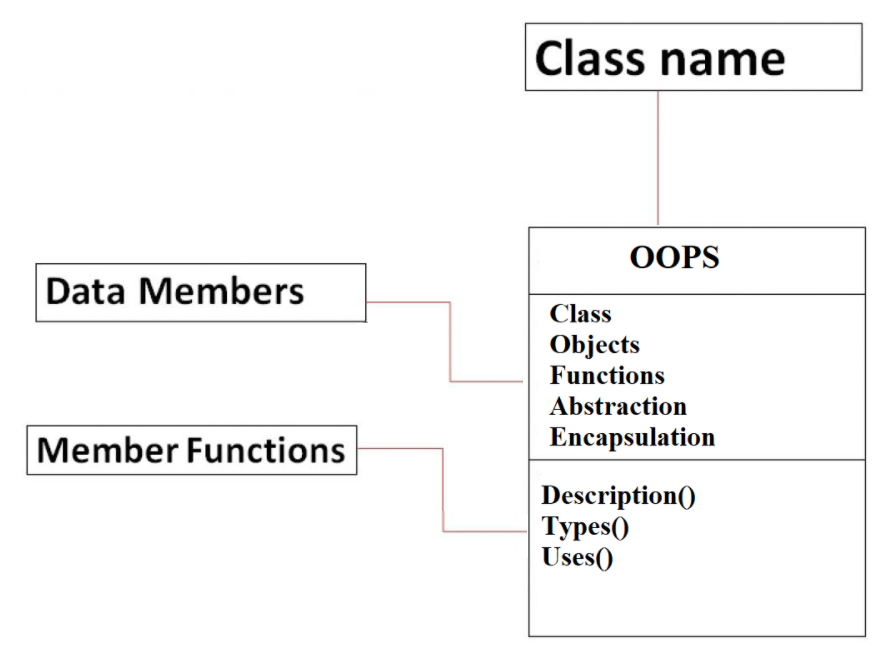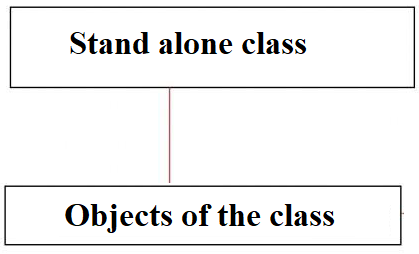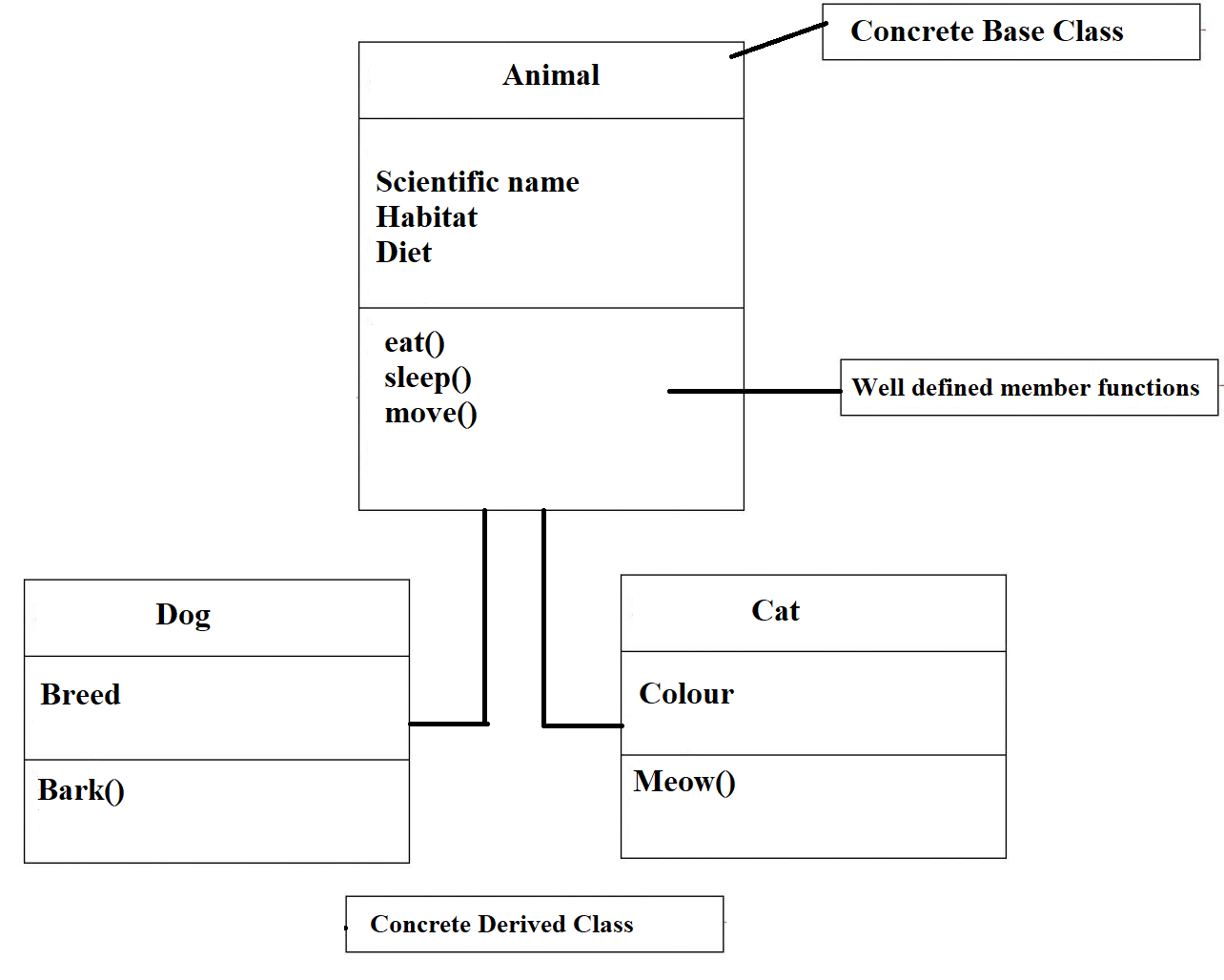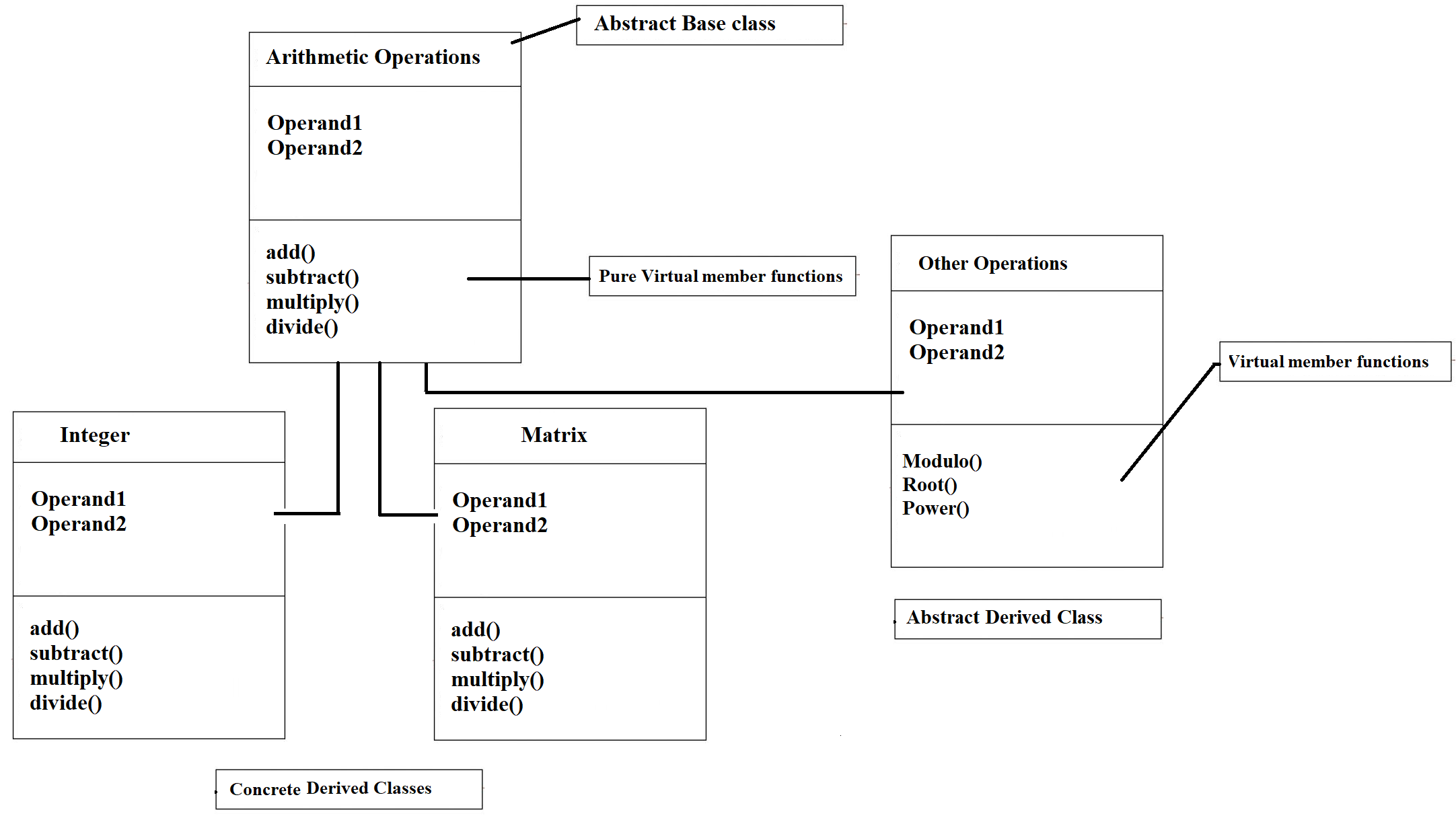×

Search anything:

# Types of classes in C++

#### Software Engineering C++We know that C++ is an object oriented programming language. An important aspect of Object-oriented programming is the usage of classes and objects. We have covered different types of classes in C++ such as Standalone classes, Abstract base class, Concrete Derived Class and much more.

1. What are classes?
2. Types of classes
3. Stand Alone Classes
4. Concrete Base Classes and Concrete Derived Classes
5. Abstract Base Classes and Abstract Derived Classes
6. Abstract base class
7. Abstract derived class
8. Friend Classes

Before we dive into the topic of discussion, let us review the basics of classes.

# What are classes?

A class is a user-defined data type. It holds its own data members and member functions, which can be accessed and used by creating an instance of that class. A class is like a blueprint for an object.``````// C++ program to demonstrate
// declaration of classes

#include <bits/stdc++.h>
using namespace std;
class OpenGenus
{
public: // Access specifier
string str;  // Data Members
{
}
};

int main() {

OpenGenus obj; // Declaring an object of class OpenGenus

obj.str = "Types Of Classes"; // Initializing data member

// accessing member function
return 0;
}
``````

# Types of classes

There are four distinct types of classes which are differentiated based on implementation. They are:

• Stand Alone Classes
• Base Classes
• Derived Classes
• Friend Classes

There are six distinct types of classes which are differentiated based on definition and use. They are:

• Stand Alone Classes
• Base Classes
• Abstract Base Class
• Concrete Base Class
• Derived Classes
• Abstract Derived Class
• Concrete Derived Class
• Friend Classes

Let us see what each type is in brief.

# Stand Alone Classes

As the name suggests, these classes are neither child classes (i.e they are not derived out of any classes) nor are they parent classes (i.e they do not act as a base class)The example given above for declaration of classes in a stand-alone class

``````// C++ program to demonstrate
// stand-alone classes

#include <bits/stdc++.h>
using namespace std;
class StandAlone
{
public: // Access specifier
string str;  // Data Members
{
cout << "This section of the article describes the: " << str;
}
};

int main() {

StandAlone obj; // Declaring an object of class OpenGenus

obj.str = "Stand alone classes"; // Initializing data member

// accessing member function
return 0;
}
``````

Output: This section of the article describes the: Stand alone classes

# Concrete Base Classes and Concrete Derived Classes

A concrete class has well defined member functions. Their functions are not virtual or pure virtual (explained later).

A concrete bases class, as the name suggests, is a class which has well defined data members and functions and acts as a base for another class to derive from. A concrete derived class is a concrete class which is derived from the existing base class. It inherits the properties of the base class.In the above diagram, notice that the base class Animal has well defined member functions ( eat(), sleep(), move() ). These functions are common to all animals. This class can also be used as a stand alone class. The concrete derived classes are dog and cat. They inherit the data members and functions of the base class animal.

An example to show concrete base and derived classes

``````#include <iostream>
using namespace std;

// Concrete Base Class
class Base {
public:
int a;
};

// Concrete Derived Class
class Derived : public Base {
public:
int b;
};

// Driver Code
int main()
{

Derived obj;// Initialise an object of derived class
obj.b = 3;// Assign value to Derived class variable
obj.a = 4; // Assign value to Base class variable via derived class
cout << "Value from derived class: "<< obj.b << endl;
cout << "Value from base class: "<< obj.a << endl;
return 0;
}
``````

Output:
Value from derived class: 3
Value from derived class: 4

Differences between Concrete Base class and Concrete Derived class

Concrete Base Class Concrete Derived class
Helps to derive or create new classes Derived out of base class
Also called parent class Also called child class
Cannot inherit properties and methods of child class Can inherit properties and methods of parent class
Can be used as stand-alone class Cannot be used as a stand-alone class

# Abstract Base Classes and Abstract Derived Classes

Before we dive into the definition of the class, let us first understand what virtual functions and pure virtual functions are:

Virtual functions

Need for virtual functions:
Consider the example given below which has a concrete base and concrete derived class:

``````#include <iostream>
using namespace std;
class A  //concrete base class
{
int x=10;
public:
void display()
{
cout << "Value of x is : " << x<<endl;
}
};
class B: public A   //concrete derived class
{
int y = 15;
public:
void display()
{
cout << "Value of y is : " <<y<<endl;
}
};
int main()
{
A* a;    //pointer of base class
B b;     //object of derived class
a = &b;
a->display();
return 0;
}
``````

In the program above, the output is: Value of x is : 10.

a is the concrete base class pointer. * a can only access the base class data members but not the members of the derived class. Although C++ permits the base pointer to point to any object derived from the base class, it cannot directly access the members of the derived class.

Therefore, there is a need for a special type of function which allows the base pointer to access the members of the derived class. this type of function is called as a virtual function.

An example for virtual functions:

``````#include <iostream>
using namespace std;
class A  //concrete base class
{
int x=10;
public:
virtual void display()
{
cout << "Value of x is : " << x<<endl;
}
};
class B: public A   //concrete derived class
{
int y = 15;
public:
void display()
{
cout << "Value of y is : " <<y<<endl;
}
};
int main()
{
A* a;    //pointer of base class
B b;     //object of derived class
a = &b;
a->display();
return 0;
}
``````

In the above example, the output is: Value of y is : 15

Pure Virtual functions

Need for pure virtual functions:

A pure virtual function (or a "do-nothing" function) is not used for performing any task.A pure virtual function is a function declared in the base class that has no definition in the base class. The below example shows a pure virtual function.

``````virtual void show() = 0;
``````

# Abstract base class

A class containing the pure virtual function cannot be used to declare the objects of its own .Such classes are known as abstract base classes.

The main objective of the abstract base class is to provide the features to the derived classes and to create the base pointer used for achieving the runtime polymorphism.

# Abstract derived class

A class which is derived from an abstract base class is called as an abstract derived class.The main objective of the abstract derived class is to inherit the features of the abstract base class. The remaining functions are all virtual functions. It is still an abstract class and hence objects of the class cannot be defined.

Note: The derived class is abstract only if its member functions are virtual.In the above diagram, arithmetic operations is an abstract base class with pure virtual functions add(), subtract(), multiply() , divide(). It references integer, matrix and other operations derived classes.

The reason for this implementation is that although we can perform the same 4 arithmetic operations on both integers and matrices, the definition for the operations differ for both classes. Since the four functions add(), subtract(), multiply() , divide() are overwritten in integers and multiply, they are concrete derived classes

In the derived class, other operations, the four base pure virtual functions are not overwritten although it is derived out of Operations class. Rather other virtual functions are introduced. Therefore Other Operations is an abstract derived class and an object of this class cannot be created.

An example to show the working of abstract classes

``````#include <iostream>
using namespace std;
class A  //abstract base class
{
public:
virtual void show() = 0;  //pure virtual function
};

class B : public A  // derived class
{
public:
void show()
{
cout << "This is the derived class" << endl;
}
};
int main()
{
A *a;
B b;
a = &b;
a->show();
return 0;
}
``````

Output: This is the derived class

Differences between Abstract Base class and Abstract Derived class

Abstract Base Class Abstract Derived class
Helps to derive or create new classes Derived out of base class
Also called parent class Also called child class
This class contains pure virtual functions This class contains virtual functions

Let us consider an example to understand the differences between abstract and concrete derived classes.

``````class abstract_base {
public:
virtual void abstract_method1() = 0;
virtual void abstract_method2() = 0;
};

class concrete_base {
public:
void concrete_method1() {
/* random code */
}
};

class abstract_derived1 : public abstract_base {
public:
virtual void abstract_method3() = 0;
};

class abstract_derived2 : public concrete_base {
public:
virtual void abstract_method3() = 0;
};

class abstract_derived3 : public abstract_base {
public:
virtual abstract_method1() {
/* random code */
}
};

class concrete_derived1 : public concrete_base {
public:
virtual void abstract_method1() {
/* random code */
}
virtual void abstract_method2() {
/* random code */
}
/* This class is now concrete because no abstract methods remain */
};

class example : public abstract_base {
public:
virtual void abstract_method2() {
/* random code */
}
};
``````

Note that we do not provide an implementation for abstract_method1 and abstract_method2, so the abstract_derived classes are still abstract and objects of this class cannot be created.

## Question

#### What type of a class is example in the above program?

Concrete Derived class
Abstract Derived class
Concrete Base Class
Abstract Base Class
Class example is a concrete derived class although it is derived from an abstract class. This is because one of the pure virtual base function is overwritten and there are no existing virtual functions within the class definition. An object of class question can be created

# Friend Classes

Need for friend classes

Data encapsulation is an important concept of object-oriented programming. It restricts the access of private members from outside of the class.

Similarly, protected members can only be accessed by derived classes and are inaccessible from outside. For example,

``````
class A {
private:
int num;
}

int main() {
A obj;
A.num = 5;// Private data members cannot be accessed from here
}
``````

Friend Functions

A friend function can access the private and protected data of a class. We declare a friend function using the friend keyword inside the body of the class. Below is an example of a friend functions

``````class className {
... .. ...
friend returnType functionName(arguments);
... .. ...
}
``````

Similar to friend functions, We can also use a friend Class in C++ using the friend keyword. For example,

``````class ClassFriend;

class ClassA {
// ClassFriend is a friend class of ClassA
friend class ClassFriend;
... .. ...
}

class ClassFriend {
... .. ...
}
``````

When a class is declared a friend class, all the member functions of the friend class become friend functions.Since ClassFriend is a friend class, we can access all members of ClassA from inside ClassFriend.

Note: We cannot access members of ClassFriend from inside ClassA. It is because friend relation in C++ is only given, not taken.

An example depicting the use of friend class

``````#include <iostream>
using namespace std;

class Square
{
protected:
int side;
friend class Rectangle;
public:

Square (int s) // parameterised constructor
{
side = s;
}
};

class Rectangle{
int length;
public:
int getArea(){
}
void shape(Square a){
length = a.side;
}
};

int main(){
Square square(5);
Rectangle rectangle;
rectangle.shape(square);
cout<<rectangle.getArea()<<endl;
return 0;
}
``````

Output: 25

In the above program, Rectangle is the friend function of the class Square. side is a protected member of the class Square. It is accessed by Rectangle. When square(5) object of class Square is created, side is initialized to 5. Then we create object rectangle of class Rectangle. It then accesses side of Square and initializes length and breadth to 5. When function getArea() is called, length * breadth = 25 is returned.

In summary, we have discussed about the different types of classes and their definitions. We have seen their uses and when to apply them. To stress again, classes are very important in object-oriented programming. If used well, the program becomes simpler and modular. Happy Coding!#### Rohan Chandrashekar

Rohan Chandrashekar is pursuing B. Tech in Computer Science at Vellore Institute of Technology (2020 to 2024) and has been an Algorithm and Data Structure Developer, Intern at OpenGenus.

Types of classes in C++